## 30 April, 2021

### SUDOKU Solver and Creator

If you are Sudoku solver you probably spent hours and hours trying to solve the puzzles. I'd like to show here that in Excel, with help of VBA macros, you can do it usually in seconds.

Create your own private Sudoku solver tool, which will not only solve the puzzle but also create your own, at different levels of difficulty. I'll guide you through all the steps.

In the first step, you'll need to design your worksheet structure/view. Start with Sudoku 9 x 9 cells square. Use any colors you like to create a pleasant display of the matrix. I've used RowHeight of 33 and ColumnWidth of 6 to make cells look like squares.

Here is my design shown in two stages: with blank fields (before creating a puzzle) and with filled fields (after solving a puzzle).

After formatting of the layout as presented in the example above, comes the second step - filling designated cells with formulas. Enter them as follows:

• Make sure that the range of cells P1:X9 is left blank.
• Hide Columns L:AJ and AN:DP.

Step three. Now you need to insert three command buttons: Create, Solve, and Clear, as you see in the example above.

In Developer Menu Controls turn on the Design Mode and click on Insert to insert Command Button from ActiveX Controls. Size the button as needed.

Right-click on the button and select Properties. Change Caption Name to "Create". Right-click again on the button and select View Code. In the Sheet1 code page enter the following code:

Private Sub CommandButton1_Click()
'CommandButton "Create"
Range("sudoku").Font.Color = vbBlack
RrLogic = 1
MainCod
End Sub

Return to your worksheet and create next two buttons the same way, but name them "Solve" and "Clear".

For "Solve" button enter the following code:

Private Sub CommandButton2_Click()
'CommandButton "Solve"
'Dim CheckArray(9)
If Cells(1, 38) = 0 Then End ' Checks if the empty cells are 0(solved sudoku)
Range("sudoku").Font.Color = vbBlack
For x = 1 To 9 'checks if there are same numbers in row,column,box
For y = 1 To 9
rX = "a" & x & ":" & "i" & x ' Finds the coords of the range(row,column,box)
rY = Chr(y + 64) & "1" & ":" & Chr(y + 64) & "9"
xK = 1 + Int((x - 1) / 3) * 3
yK = 1 + Int((y - 1) / 3) * 3
rK = Chr(yK + 64) & xK & ":" & Chr(yK + 66) & xK + 2
r = rX & "," & rY & "," & rK
Set MyRange = Range(r)
If Cells(x, y) = "" Then GoTo nextxy
temp = Cells(x, y)
Cells(x, y) = ""
For Each c In MyRange.Cells
If c.Value = temp Then
Cells(x, y) = temp
c.Font.Color = vbRed
Cells(x, y).Font.Color = vbRed
Message = MsgBox("Exits Sudoku", vbOKOnly)
End
End If
Next
Cells(x, y) = temp
nextxy:
Next y, x
For x = 1 To 9 'Input data in an array
For y = 1 To 9
InputData(x, y) = Cells(x, y)
Next y, x
RrLogic = 2 ' If RrLogic =1 then Create Sudoku or If RrLogic =2 then Solve Sudoku
MainCod
End Sub

For "Clear" button enter this code:

Private Sub CommandButton3_Click()
'CommandButton "Clear"
Range("sudoku").Font.Color = vbBlack
For x = 1 To 9
For y = 1 To 9
Cells(x, y) = ""
Next y, x
End Sub

In step four, enter shortcut ALT+F11 to open VBE page and click on the View in menu to select Project Explorer. If there is no Module available within your VBA Project, then insert one by clicking on the Insert in the menu. Double-click on the Module and and paste there the following Sub procedures:

Public InputData(9, 9), SpecialAr(), XCoords(), YCoords(), RrCount, RrLogic, CurNum As Integer
Public IsZero, AnyChanges As Boolean
Public x, y, MidNum, TempVar
Public MyRange As Range
Public TheSame As String

Public Sub MainCod()
Randomize Timer
wrongRep = 0: Xronos = Timer
1 IsZero = False
If RrLogic = 1 Then CreateSudoku Else SolveSudoku
wrongRep = wrongRep + 1     'Count of tries to solve sudoku
Do
CheckArea:
IsZero = False      'Becomes True when "123456789" is zero
If Cells(2, 38) = 1 Then GoTo 3 'Check if unique num in 2nd string array (in row, col or box)
ReDim SpecialAr(9), XCoords(4), YCoords(4)
AnyChanges = False  'True if any changes made after any check
For x = 1 To 9  'Row check
For y = 1 To 9
For k = 1 To Len(Cells(x, y + 15))
If Cells(x, y + 15) = "0000000000" Then Exit For
MidNum = Mid(Cells(x, y + 15), k, 1)
SpecialAr(MidNum) = SpecialAr(MidNum) + 1     'Counts occurrence of a number in row
Next k
Next y
For l = 1 To 9
If SpecialAr(l) = 1 Then
For y = 1 To 9      'Find cell with the unique number
For k = 1 To Len(Cells(x, y + 15))     'Loop every char in the string
MidNum = Mid(Cells(x, y + 15), k, 1)
If Val(MidNum) = l Then
Cells(x, y) = l     'The unique number in the 1st table
Cells(x, y + 15) = "0000000000"     'Set the string in the array for every completed cell in the 1st table
CurNum = Cells(x, y)     'Current cell investigated
RepairStr   'Calls the sub fixing the string table after cell is completed in 1st table
If IsZero = True Then GoTo 1 'Go back to the beginning if cannot go any further
GoTo CheckArea      'Make all the check if any change has been made
End If
Next k, y
Else
End If
Next l
ReDim SpecialAr(9)
Next x
'Column check
For y = 1 To 9     'The same as above with exchanging the x and y coordinates
For x = 1 To 9
For k = 1 To Len(Cells(x, y + 15))
If Cells(x, y + 15) = "0000000000" Then Exit For
MidNum = Mid(Cells(x, y + 15), k, 1)
SpecialAr(MidNum) = SpecialAr(MidNum) + 1
Next k
Next x
For l = 1 To 9
If SpecialAr(l) = 1 Then
For x = 1 To 9
For k = 1 To Len(Cells(x, y + 15))
MidNum = Mid(Cells(x, y + 15), k, 1)
If Val(MidNum) = l Then
Cells(x, y) = l: Cells(x, y + 15) = "0000000000"
CurNum = Cells(x, y)
RepairStr
If IsZero = True Then GoTo 1
GoTo CheckArea
End If
Next k, x
Else
End If
Next l
ReDim SpecialAr(9)
Next y
x = 0: y = 0
'Box check
For i = 1 To 6 Step 3     'The same as above but using the x and y coordinates of the box
For j = 1 To 6 Step 3
For n = 0 To 2
For g = 0 To 2
x = i + n: y = j + g
For k = 1 To Len(Cells(x, y + 15))
If Cells(x, y + 15) = "0000000000" Then Exit For
MidNum = Mid(Cells(x, y + 15), k, 1)
SpecialAr(MidNum) = SpecialAr(MidNum) + 1
Next k
Next g, n
For l = 1 To 9
If SpecialAr(l) = 1 Then
For n = 0 To 2
For g = 0 To 2
x = i + n: y = j + g
For k = 1 To Len(Cells(x, y + 15))
MidNum = Mid(Cells(x, y + 15), k, 1)
If Val(MidNum) = l Then
Cells(x, y) = l: Cells(x, y + 15) = "0000000000"
CurNum = Cells(x, y)
RepairStr
If IsZero = True Then GoTo 1
GoTo CheckArea
End If
Next k
Next g, n
Else
End If
Next l
ReDim SpecialAr(9)Next j, i
'Check if there is a couple a triad or tetrad in the same row, column or box
If RrLogic = 1 Then GoTo 3
For x = 1 To 9 'row check
For y = 1 To 8
For k = y + 1 To 9
If Cells(x, y + 15) = Cells(x, k + 15) And Len(Cells(x, y + 15)) < 5 Then
YCoords(1) = y: TheSame = Cells(x, y + 15)
For i = 2 To 4     'Give the Y coords of the same cells to the YCoords array
If YCoords(i) = 0 Then YCoords(i) = k: Exit For
Next i
Else
End If
Next k
'If there are same cells, call the CheckSameY sub & keep the current row in the TempVar variable
If YCoords(1) <> 0 Then TempVar = x:  CheckSameY: Exit For
Next y
If IsZero = True Then GoTo 1
If AnyChanges = True Then GoTo CheckArea
Next x
IsZero = False
For y = 1 To 9  'Column check
For x = 1 To 8
For k = x + 1 To 9
If Cells(x, y + 15) = Cells(k, y + 15) And Len(Cells(x, y + 15)) < 5 Then
XCoords(1) = x: TheSame = Cells(x, y + 15)
For i = 2 To 4      'Give the Y coords of the sam cells to the YCoords array
If XCoords(i) = 0 Then XCoords(i) = k: Exit For
Next i
Else
End If
Next k
'If there are same cells then call the CheckSameY sub & keep the current row in the TempVar variable
If XCoords(1) <> 0 Then TempVar = y:  RemRepeats: Exit For
Next x
If IsZero = True Then GoTo 1
If AnyChanges = True Then GoTo CheckArea
Next y
'Take the returned value of match function which finds the string with the minimum legth
3 synt = Cells(3, 38)
'Convert the coordinates of the 1x81 array to the 9x9 array
x = Int((synt - 1) / 9) + 1
y = synt - (Int((synt - 1) / 9) * 9)
PithNum = Cells(x, y + 15)     'Give to a var the string with possible number for the cell
'Return a random number from 1 to the length of the string
RndNum = Int(Rnd * Len(Cells(x, y + 15))) + 1
'Give to a var the corresponding number
CurNum = Mid(PithNum, RndNum, 1)
Cells(x, y) = CurNum
Cells(x, y + 15) = "0000000000"
RepairStr
If IsZero = True Then GoTo 1
Loop Until Cells(1, 38) = 0
Cells(4, 38) = wrongRep
Cells(5, 38) = Timer - Xronos
If RrLogic = 1 Then DelCells
End Sub

Public Sub CreateSudoku()
'Prepare the tables to start creating a new sudoku
For x = 1 To 9
For y = 1 To 9
Cells(x, y + 15) = "123456789"
Cells(x, y) = ""
Next y, x
End Sub

Public Sub SolveSudoku()
'Solve a sudoku given by the user or created from the program
For x = 1 To 9     'Gives all the combinations to the string table
For y = 1 To 9
Cells(x, y + 15) = "123456789"
Next y, x
For x = 1 To 9  'Insert all the given data from the array into the 1st table
For y = 1 To 9
If InputData(x, y) = 0 Then Cells(x, y) = "": GoTo 2
Cells(x, y) = InputData(x, y)
Cells(x, y).Font.Color = vbRed     'Cells with data become red
CurNum = Cells(x, y)     'For any given num fix the corresponding string
Cells(x, y + 15) = "0000000000"
RepairStr
If IsZero = True Then Message = MsgBox("Not Possible", vbOKOnly): End
2 Next y, x
End Sub

Public Sub RepairStr()
rX = "p" & x & ":" & "x" & x     'Make the range in 2nd table, for every cell of 1st table, which contains row, column and box
rY = Chr(y + 79) & "1" & ":" & Chr(y + 79) & "9"
xK = 1 + Int((x - 1) / 3) * 3
yK = 1 + Int((y - 1) / 3) * 3
rK = Chr(yK + 79) & xK & ":" & Chr(yK + 81) & xK + 2
r = rX & "," & rY & "," & rK
Set MyRange = Range(r)
For Each c In MyRange.Cells 'Subtract the current number from any string of the range
For j = 1 To Len(c.Value)
If Mid(c.Value, j, 1) <> CurNum Then tempXY\$ = tempXY\$ + Mid(c.Value, j, 1)
Next
If tempXY\$ = "" Then IsZero = True 'Check if the string with possible num is nothing that means that the sudoku cannot be solved
c.Value = tempXY\$
tempXY\$ = ""
Next
End Sub

Public Sub CheckSameY()
AnyChanges = False
x = TempVar: TempVar = ""     'Variable containing the current x coordinate
metritis = 0
If YCoords(Len(TheSame)) = 0 Then ReDim YCoords(4): Exit Sub

For k = 1 To 9
If Cells(x, k + 15) = "0000000000" Then metritis = metritis + 1
Next k
'Check if there are no more empty cells in the 1st table except the diads, triads or tetrads
If Len(TheSame) + metritis = 9 Then ReDim YCoords(4): Exit Sub
For y = 1 To 9
For i = 1 To Len(TheSame)
If y = YCoords(i) Then GoTo 1     'Prevent the program from subtracting the numbers from the cells which contains the diads, triads or tetrads
Next i
For i = 1 To Len(TheSame)
For k = 1 To Len(Cells(x, y + 15))
MidNum = Mid(Cells(x, y + 15), k, 1)
If MidNum <> Mid(TheSame, i, 1) Then TempVar = TempVar + MidNum
If MidNum = Mid(TheSame, i, 1) Then AnyChanges = True
Next k
If TempVar = "" Then IsZero = True
Cells(x, y + 15) = TempVar
TempVar = ""
Next i
1 Next y
ReDim YCoords(4)
End Sub
AnyChanges = False
y = TempVar: TempVar = ""     'Variable containing the current y coordinate
metritis = 0
If XCoords(Len(TheSame)) = 0 Then ReDim XCoords(4): Exit Sub
For k = 1 To 9
If Cells(k, y + 15) = "0000000000" Then metritis = metritis + 1
Next k
'Check if there are no more empty cells in the s1 tables except the diads, triads or tetrads
If Len(TheSame) + metritis = 9 Then ReDim XCoords(4): Exit Sub
For x = 1 To 9
'Prevent the program from subtracting the numbers from the cells which contains the diads, triads or tetrads
For i = 1 To Len(TheSame)
If x = XCoords(i) Then GoTo 1
Next i
For i = 1 To Len(TheSame)
For k = 1 To Len(Cells(x, y + 15))
MidNum = Mid(Cells(x, y + 15), k, 1)
If MidNum <> Mid(TheSame, i, 1) Then TempVar = TempVar + MidNum
If MidNum = Mid(TheSame, i, 1) Then AnyChanges = True
Next k
If TempVar = "" Then IsZero = True
Cells(x, y + 15) = TempVar
TempVar = ""
Next i
1 Next x
ReDim XCoords(4)
End Sub

Public Sub DelCells()
'Delete cells randomly(get ready to play)
RrCount = 0
10 Do
x = Int((Rnd * 9) + 1)
y = Int((Rnd * 9) + 1)
'Stop
If Cells(x, y) <> "" Then
Cells(x, y) = ""
Else
GoTo 10
End If
RrCount = RrCount + 1
Loop Until RrCount = Cells(6, 38)
End Sub

Go back to your worksheet. Turn off the Design Mode and check if everything was entered correctly and works as intended. Finish any formatting and save your workbook as Macro-Enabled one.

Happy Sudoku-ing...

## 29 April, 2021

### CHARTS: How to plot nice chart for any formula / equation

Would you like to create impressive charts effortlessly, based just on a single formula? Would you like your charts look similar to these examples? If so, follow the directions below.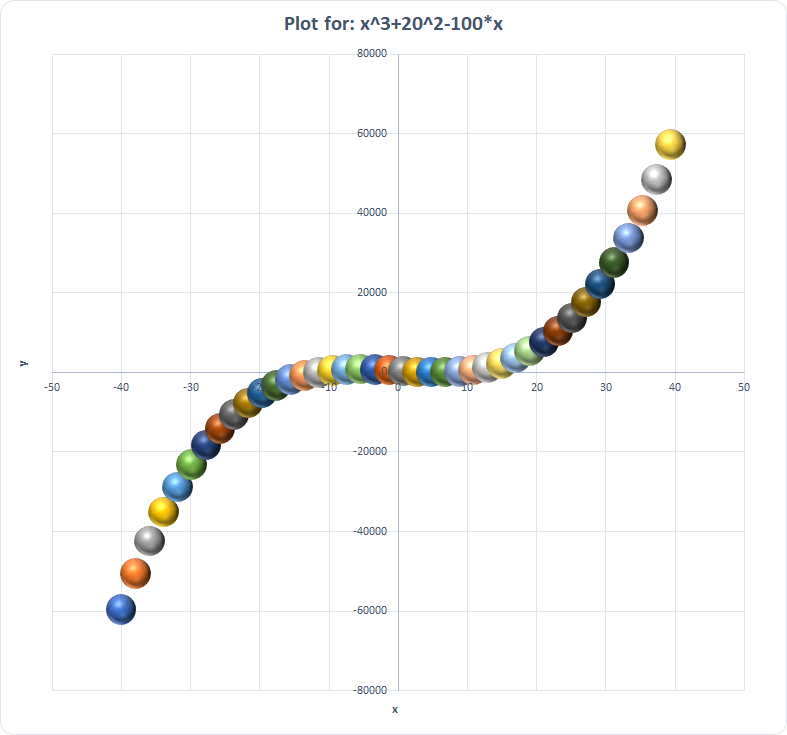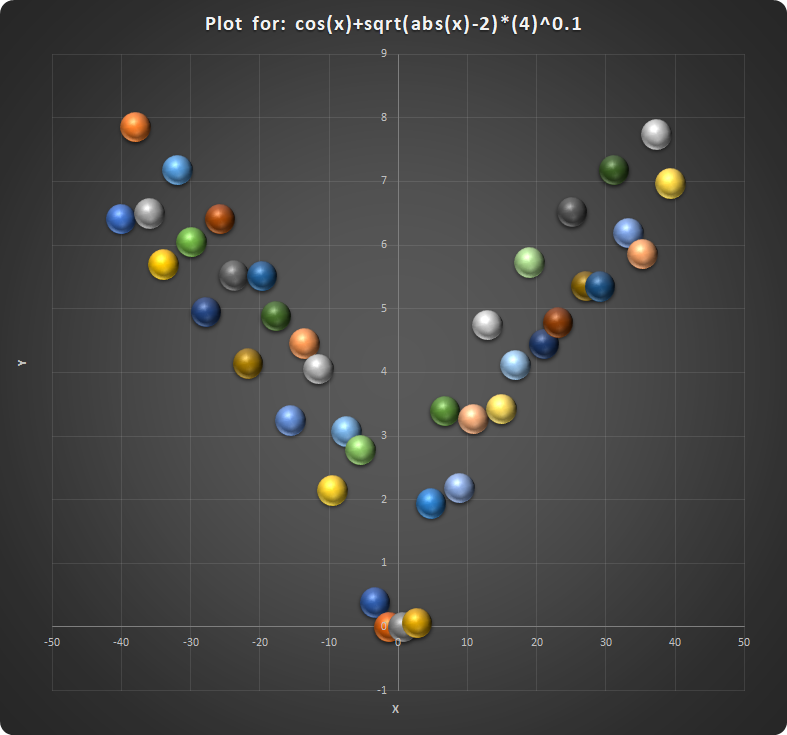One of interesting features related to charting in Excel allows you to plot charts based not on actual data ranges/tables but based on formulas.

Here are the basic steps to follow if you'd like to create such nice charts quickly. If you create just one of them, you can save the file as a template for future use.

The first thing to do is to enter/arrange all necessary information you'll need for your chart elements. So, enter the following in your worksheet:

Cell

Text entry

Cell

Data entry

A1

“Formula to plot”

B1

Enter/paste your formula to be plotted

A2

“xLeft”

B2

Enter number for starting point of X axis

A3

“xRight”

B3

Enter number for ending point of X axis

A4

“Number of points”

B4

Enter number of points to be plotted

A5

“Chart title”

B5

Enter Plott for: “Sheet1!\$B\$1

A7

List of formulas

A8

Enter your first formula, e.g. x^3+20^2-100*x

A9

and 2nd one, e.g.

 (sqrt(cos(x))*cos(500*x)+sqrt(abs(x))-0.4)*(4-x*x)

A10

Etc….

When you enter all required information your worksheet may look similar to this one:

Remember that you have to follow the format of the formulas exactly as shown here in the examples. No free spaces are allowed within the equation; they would create error message. Remember also that you can change the plot view by changing coordinates and number of points plotted (entries in cells B2:B4).

The second thing to do is to define some Names. On Formulas tab, in "Define Names group, click "Define Name" and define the following Names, one by one:

 Name Refers To: Formula =Sheet1!\$B\$1 xLeft =Sheet1!\$B\$2 xRight =Sheet1!\$B\$3 xNoOfPoints =Sheet1!\$B\$4 xRng =xRight-xLeft x =xLeft+xRng/(xNoOfPoints-1)*(ROW(OFFSET(Sheet1!\$A\$1,0,0,xNoOfPoints,1))-1) y =EVALUATE(Sheet1!\$B\$1 & ”+0*x”

Now, select any area in your worksheet and, in your the Menu Bar select Insert>Charts>Insert Scatter(X,Y)... Select one of Scatter charts. Place the chart conveniently in your worksheet and right-click on the chart area. In Edit Series window, click on Edit button and in Edit Series window enter:

• under Series name: select range B5 in the worksheet,
• under Series X values: enter "=Shee1!x"
• under Series Y values: enter "=Sheet1!y"

Now you can format your data points in the chart. Right-click in chart area and select Change Chart Type. Select All Charts and click on 3-D Bubble icon. Click OK.

Next, right-click on data points (markers) and select Format Data Series... . Click on Series Options icon (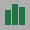) , set the Scale bubble size to e.g. 15 (Area of bubbles), then click on Fill & Line icon (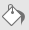), select Fill>Automatic and mark Vary colors by point.

If you need to edit/add chart title, right-click on the title box and either:

• select Edit Text and edit it, or
• in Formula Bar enter "=" and select cell B5.

Select the chart, click on Chart Elements icon (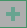) and select those elements which you want to be displayed in the chart (titles, axes, gridlines, data labels, error bars, legend, trendline). Make any changes you want to those elements.

Select the chart and click on Chart Styles icon (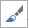) and select your favorite style and color scheme for your chart.

Have you got any interesting chart? If so, please share it with me.